• 最优化方法课件，杨振华老师的课件，绝对精品，吐血推荐
• 实用最优化方法实用最实用最优化方法优化方法实用最优化方法实用最优化方法
http://blog.csdn.net/pipisorry/article/details/52135832

深度学习最优化算法

深度学习框架中常见的最优化方法。

tensorflow tf.keras.optimizers中的最优化方法及相关参数如下：

[https://www.tensorflow.org/api_docs/python/tf/keras/optimizers][tensorflow Optimizers]

pytorch中的最优化方法及相关参数如下：

[https://pytorch.org/docs/stable/optim.html#]

基本算法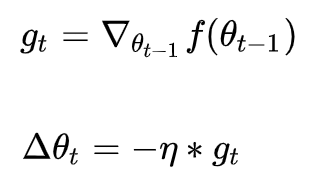θ^t+1 = θ^t + delta(θ^t)

其中，η是学习率，g_t是梯度SGD完全依赖于当前batch的梯度。

缺点：SGD 的缺点在于收敛速度慢，可能在鞍点处震荡，并且如何合理的选择学习率是 SGD 的一大难点。

具体为1 选择合适的learning rate比较困难- 对所有的参数更新使用同样的learning rate。对于稀疏数据或者特征，有时我们可能想更新快一些（lz稀疏特征少，不平滑，影响更大），对于不经常出现的特征，对于常出现的特征更新慢一些，这时候SGD就不太能满足要求了。2 SGD容易收敛到局部最优，并且在某些情况下可能被困在鞍点【查阅论文发现，其实在合适的初始化和step size的情况下，鞍点的影响并没这么大】。

[最优化方法：梯度下降（批梯度下降和随机梯度下降）]

动量Momentum

如果把要优化的目标函数看成山谷的话，可以把要优化的参数看成滚下山的石头，参数随机化为一个随机数可以看做在山谷的某个位置以0速度开始往下滚。目标函数的梯度可以看做给石头施加的力，由力学定律知：F=m∗a，所以梯度（施加的力）与石头下滚的加速度成正比。因而，梯度直接影响速度，速度的累加得到石头的位置，对这个物理过程进行建模，可以得到参数更新过程为：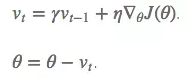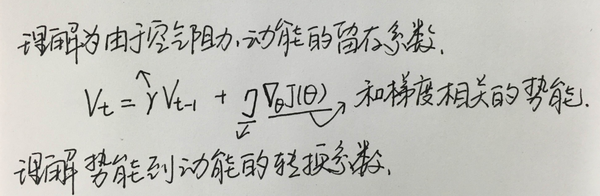启发式算法：物体的动能 = 上一个时刻的动能 + 上一时刻的势能差（相对于单位质量），当前的速度取决于上一个时刻的速度和势能的改变量。由于有阻力和转换时的损失，所以两者都乘以一个系数。

这样在更新参数时，除了考虑到梯度以外，还考虑了上一个时刻参数的历史变更幅度。如果参数上一次更新幅度较大，并且梯度还不小（当前还远未到达谷底），那么再更新时也应该很猛烈（不一定更猛烈，因为还有系数嘛，但是正因为有系数，不好定，所以不怎么自适应）。

# Momentum update
v = momentum * v - learning_rate * dx # integrate velocity
x += v # integrate position

代码中v指代速度，其计算过程中有一个超参数momentum，称为动量（momentum）。虽然名字为动量，其物理意义更接近于摩擦，其可以降低速度值，降低了系统的动能，防止石头在山谷的最底部不能停止情况的发生（除非momentum为0，不然还是停不下来的，可能通过NAG解决）。

动量的取值范围通常为[0.5, 0.9, 0.95, 0.99]，一种常见的做法是在迭代开始时将其设为0.5，在一定的迭代次数（epoch）后，将其值更新为0.99。

在实践中，一般采用SGD+momentum的配置，相比普通的SGD方法，这种配置通常能极大地加快收敛速度。faster convergence and reduced oscillation振荡。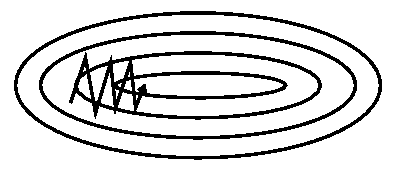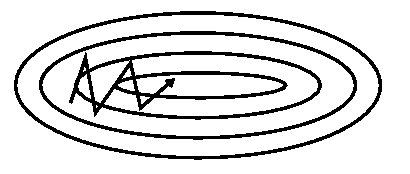还是以小球的例子来看，momentum方式有个缺点就是在临近最优点附近控制不住速度（梯度为0了，但是moment不为0，还是有速度，还会运动；lz并且快到最优点时，势能系数*势能还是>0的，还会运动）。我们希望小球很smart，它可以预判后面的"地形"，要是后面地形还是很陡，那就继续坚定不移的大胆走下去；不然的话咱就收敛点。当然，小球自己也不知道真正要走到哪里，所以这里以(θ−γv_{t−1})作为近似下一位置。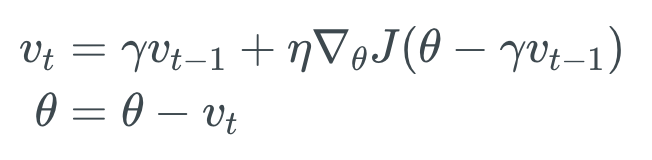Hinton的slides是这样给出的：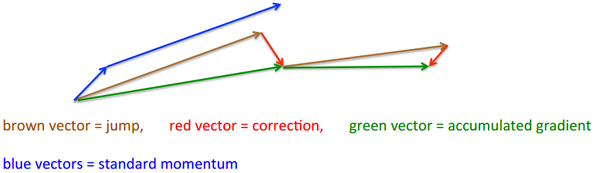momentum首先计算一个梯度(短的蓝色向量，势能)，然后在加速更新梯度的方向进行一个大的跳跃(长的蓝色向量，动能)；两个blue vectors分别理解为梯度和动能，两个向量和即为momentum方式的作用结果。

nesterov项首先在之前加速的梯度方向进行一个大的跳跃(棕色向量，动能)，计算梯度然后进行校正(绿色梯向量，势能)（反着来也没问题）；靠左边的brown vector是动能，可以看出它那条blue vector是平行的，但是它预测了下一阶段的梯度是red vector，因此向量和就是green vector，即NAG方式的作用结果。

-柚子皮-

其实，momentum项和nesterov项都是为了使梯度更新更加灵活，对不同情况有针对性。但是，人工设置一些学习率总还是有些生硬（之前所讲的方法中所有参数在更新时均使用同一个learning rate），接下来介绍几种自适应学习率的方法。

自适应学习率算法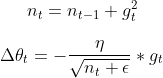（lz一点直观上看，当前梯度g_t在历史梯度和中占比大则更新快点）

对迭代次数 t，基于每个参数之前计算的梯度值，将每个参数的学习率η按如下方式修正：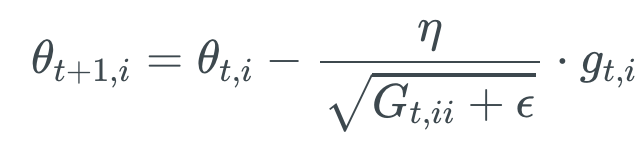其中G是一个对角阵，对角线上的元素是从一开始到 t 时刻目标函数对于参数梯度的平方和；\epsilon是一个平滑项，以避免分母为 0 的情况，它的数量级通常在1e^-8；一般超参数 η 就取 0.01。有趣的是，如果不开方的话，这个算法的表现会变得很糟。

因为在其对角线上，含有过去目标函数对于参数 梯度的平方和，我们可以利用一个元素对元素的向量乘法，将我们的表达式向量化：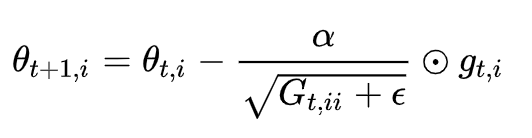[深度解读最流行的优化算法：梯度下降]

特点：

前期g_t较小的时候， regularizer较大，能够放大梯度；后期g_t较大的时候，regularizer较小，能够约束梯度；
主要优势之一，是它不需要对每个学习率手工地调节。
适合处理稀疏梯度。（"It adapts the learning rate to the parameters, performing larger updates for infrequent and smaller updates for frequent parameters." 它对不同的参数调整学习率，具体而言，对低频出现的参数进行大的更新（lz因为累积和小），对高频出现的参数进行小的更新。因此，他很适合于处理稀疏数据，对于稀疏数据上有着良好的表现。）
缺点：

由公式可以看出，仍依赖于人工设置一个全局学习率
η设置过大的话，会使regularizer过于敏感，对梯度的调节太大
然而一般大多数自适应的learning rate都是有根据变化幅度而更改，并且具有逐渐收敛的特性。所以这种启发式方法仅是一种可行性方式，并不是唯一的，也绝不是最好的，把握其本质即可。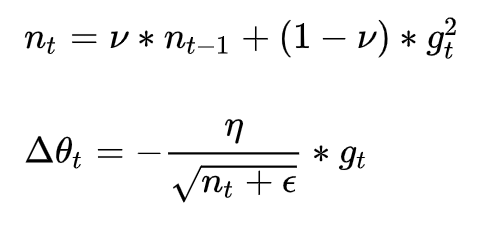超参数设定值: 学习率 η 为 0.001。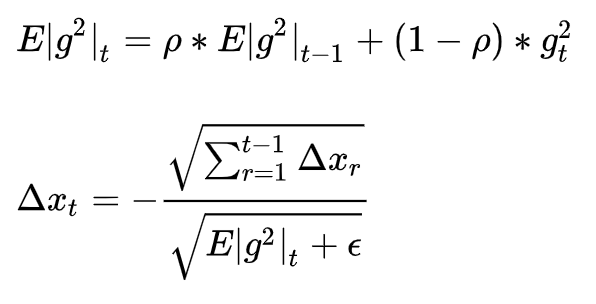其中，超参数一般设定为 0.9；E代表求期望。

因为分母表达式的形式与梯度值的方均根（root mean squared，RMS）形式类似，因而我们使用相应的简写来替换：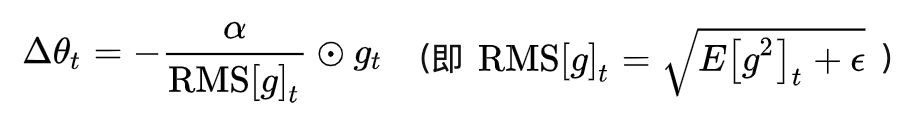特点：

训练初中期，加速效果不错，很快
训练后期，反复在局部最小值附近抖动
RMSprop

当\rho=0.5时，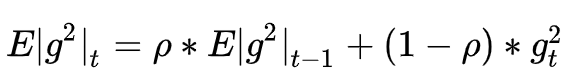就变为了求梯度平方和的平均数。

（注：Hinton建议设定 \rho 为0.9，对\eta  而言，0.001是一个较好的默认值。）

如果再求根的话，就变成了RMS(均方根)。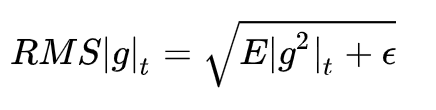此时，这个RMS就可以作为学习率\eta的一个约束：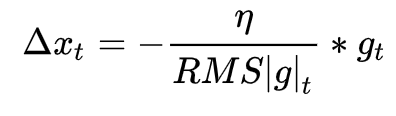特点：

其实RMSprop依然依赖于全局学习率
适合处理非平稳目标- 对于RNN效果很好

除了像 Adadelta 和 RMSprop 一样存储了过去梯度的平方 vt 的指数衰减平均值 ，也像 momentum 一样保持了过去梯度 mt 的指数衰减平均值：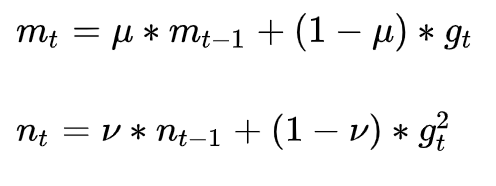m_t、n_t分别是对梯度的一阶矩估计和二阶矩估计，可以看作对期望E|g_t|，E|g_t^2|的估计。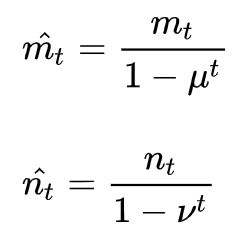\hat{m_t}，\hat{n_t}是对m_t、n_t的校正，这样可以近似为对期望的无偏估计。如果 mt 和 vt 被初始化为 0 向量，那它们就会向 0 偏置，所以做了偏差校正， 通过计算偏差校正后的 mt 和 vt 来抵消这些偏差。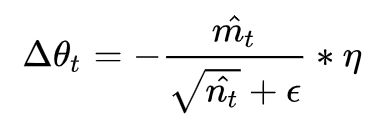可以看出，直接对梯度的矩估计对内存没有额外的要求，而且可以根据梯度进行动态调整，而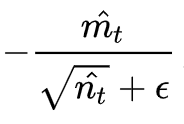对学习率形成一个动态约束，而且有明确的范围。

超参数设定值: 建议 u＝ 0.9，v ＝ 0.999，ϵ ＝ 10e−8。

特点：

对内存需求较小
为不同的参数计算不同的自适应学习率
也适用于大多非凸优化- 适用于大数据集和高维空间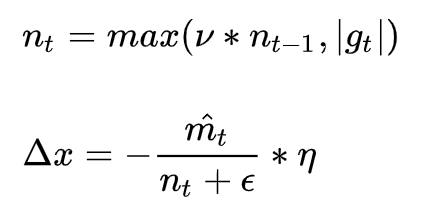公式如下：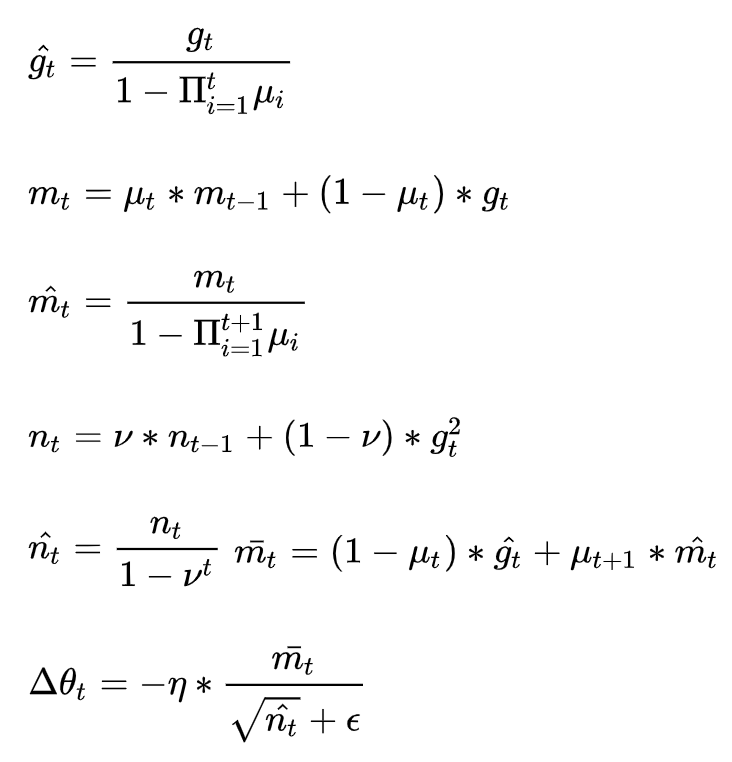-柚子皮-

不同最优化算法对比

经验之谈

对于稀疏数据，尽量使用学习率可自适应的优化方法，不用手动调节，而且最好采用默认值。
SGD通常训练时间更长，但是在好的初始化和学习率调度方案的情况下，结果更可靠。
如果在意更快的收敛，并且需要训练较深较复杂的网络时，推荐使用学习率自适应的优化方法。

如果需要更快的收敛，或者是训练更深更复杂的神经网络，需要用一种自适应的算法。

学习速率 alpha

如果 alpha 设置得非常大，那么训练可能不会收敛，就直接发散了；
如果 alpha 设置的比较小，虽然可以收敛，但是训练时间可能无法接受；
如果 alpha 设置的稍微高一些，训练速度会很快，但是当接近最优点会发生震荡，甚至无法稳定。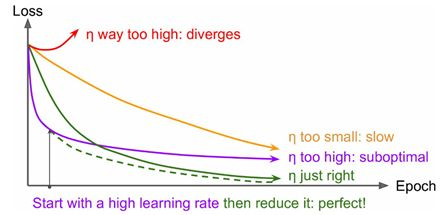不同学习速率的训练效果

理想的学习速率是：刚开始设置较大，有很快的收敛速度，然后慢慢衰减，保证稳定到达最优点。所以，前面的很多算法都是学习速率自适应的。

效果比较

几种最优化算法在“鞍点”（optimization on saddle point）和“损失平面等高线”（optimization on loss surface contours）上的表现：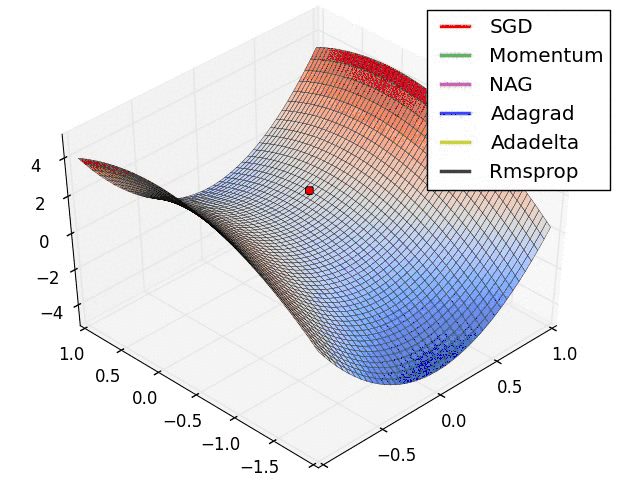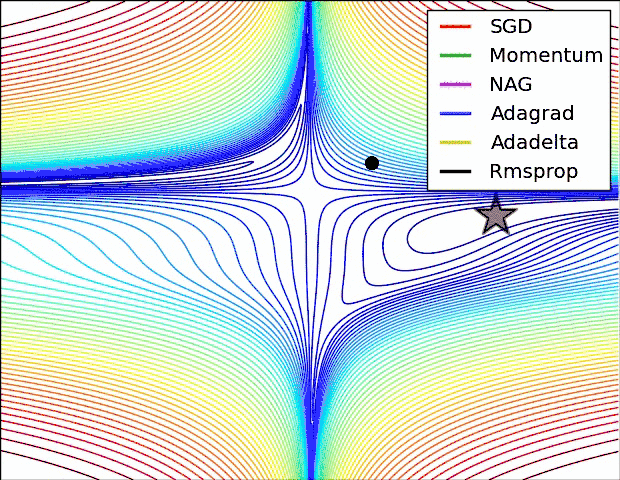[深度学习优化器算法详解：梯度更新规则+缺点+如何选择]

from: http://blog.csdn.net/pipisorry/article/details/52135832

[David Silver 增强学习补充知识——梯度下降法]*

[深度解读最流行的优化算法：梯度下降]


展开全文深度学习
• ## MATLAB最优化方法

千人学习 2019-05-14 21:54:51
结合实例介绍MATLAB最优化工具箱的主要功能。matlab matlab安装教程 matlab下载 matlab图像处理
• 最优化方法及MATLAB的实现的指导书，很有用，pdf，最优化方法及MATLAB的实现（最优化技术方法及MATLAB的实现）matlab
• 最优化方法与最优控制（研究生用）第一章最优化方法的一般概念第二章非线性规划第三章线性规划第四章最优控制与变分法第五章最小值定理第六章线性二次型最优控制系统第七章动态规划习题
• 最优化方法第二版 施光燕主编 课后习题答案 中国高等教育出版社出版
• 最优化方法及其应用/郭科，陈聆，魏友华.-北京：高等教育出版社,2007.7(2013.7重印) 前面我们已经讨论无约束问题的最优化方法，但实际碰到的问题常常是存在约束的。一般的约束最优化问题的数学模型为： minf(X)s....
主要参考书目：

最优化方法及其应用/郭科，陈聆，魏友华.-北京：高等教育出版社,2007.7(2013.7重印)

前面我们已经讨论无约束问题的最优化方法，但实际碰到的问题常常是存在约束的。一般的约束最优化问题的数学模型为：
minf(X)s.t.{gi(X)≥0,  i=1,2,⋯,l,hj(X)=0,  j=1,2,⋯,m.
minf(X)\\
s.t.\left\{\begin{matrix}
g_i (X) \ge 0,\ \ i=1,2,\cdots,l,\\
h_j (X) = 0,\ \ j=1,2,\cdots,m.
\end{matrix}\right.

解决约束问题一般有两种思路，一是通过“罚函数”把约束问题转化为无约束问题解决；二则是构造某种迭代过程使之不能越出可行域。

1、外点罚函数法

基本思路
构造一函数为
F(X,Mk)=f(X)+Mkα(X),$F\left(X,{M}_{k}\right)=f\left(X\right)+{M}_{k}\alpha \left(X\right),$
F(X,M_k)=f(X)+M_k\alpha (X),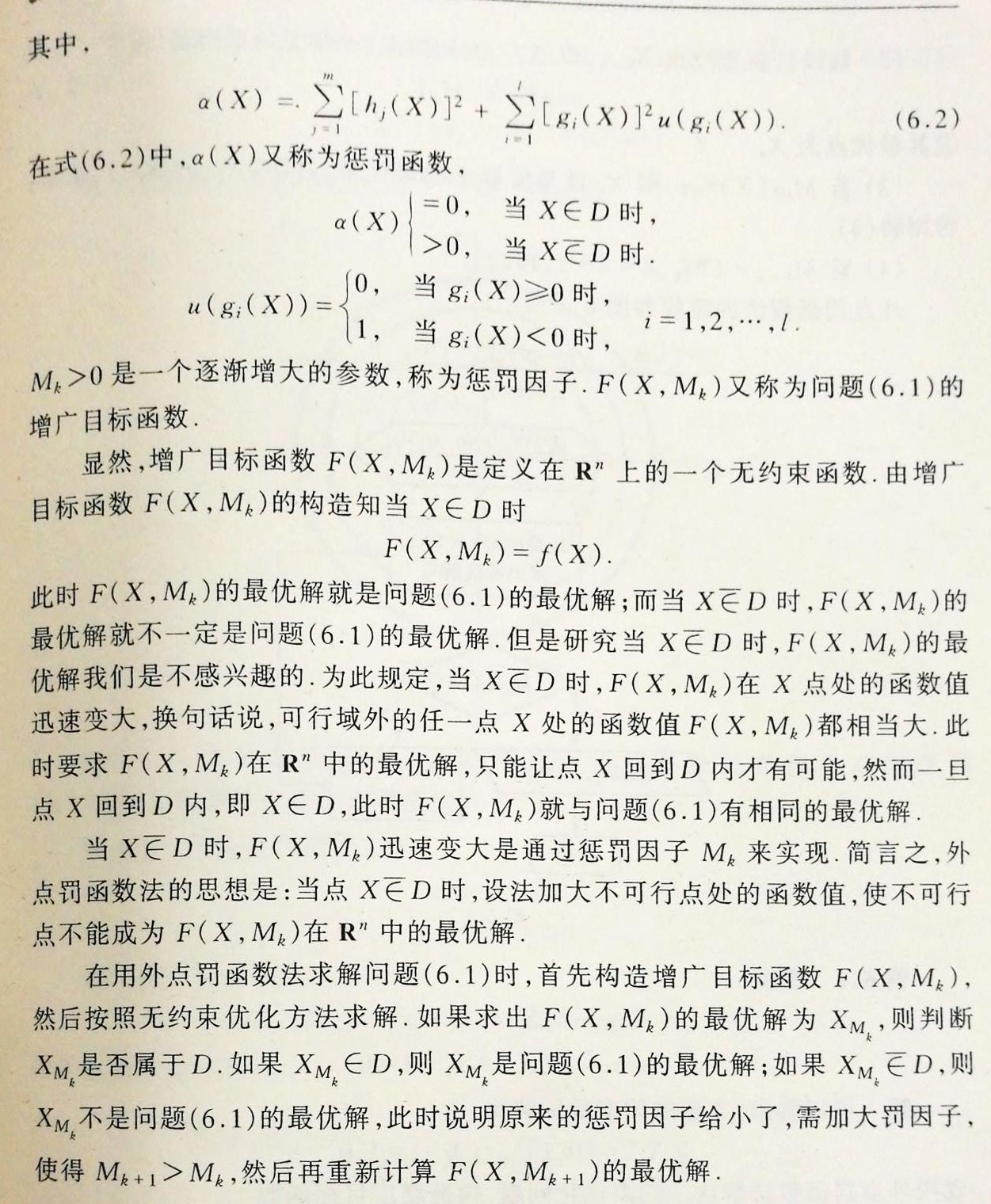该方法之所以叫做外点法，是因为在很多情况下（不是所有情况），当惩罚因子比较小的时候，最优解会落在可行域之外，随着惩罚因子的增加，向可行域内部移动，也就是说迭代过程中最优解一直在可行域之外。
特点
该方法实现了有约束问题向无约束问题的转化，但存在着一些问题：
(1)惩罚因子选的小会使最优解更容易落在可行域外，而惩罚因子选的大又会使函数的Hesse矩阵性质变坏。
(2)迭代过程中得到的解常常在可行域之外，难以观察到内点的变化情况也无法求得近似最优解。

2、内点罚函数法

基本思路
对于纯不等式约束问题，为使得迭代过程中解一直在可行域内。我们通过罚函数在可行域边界处构造一个“壁垒”使得迭代过程中解不能离开可行域。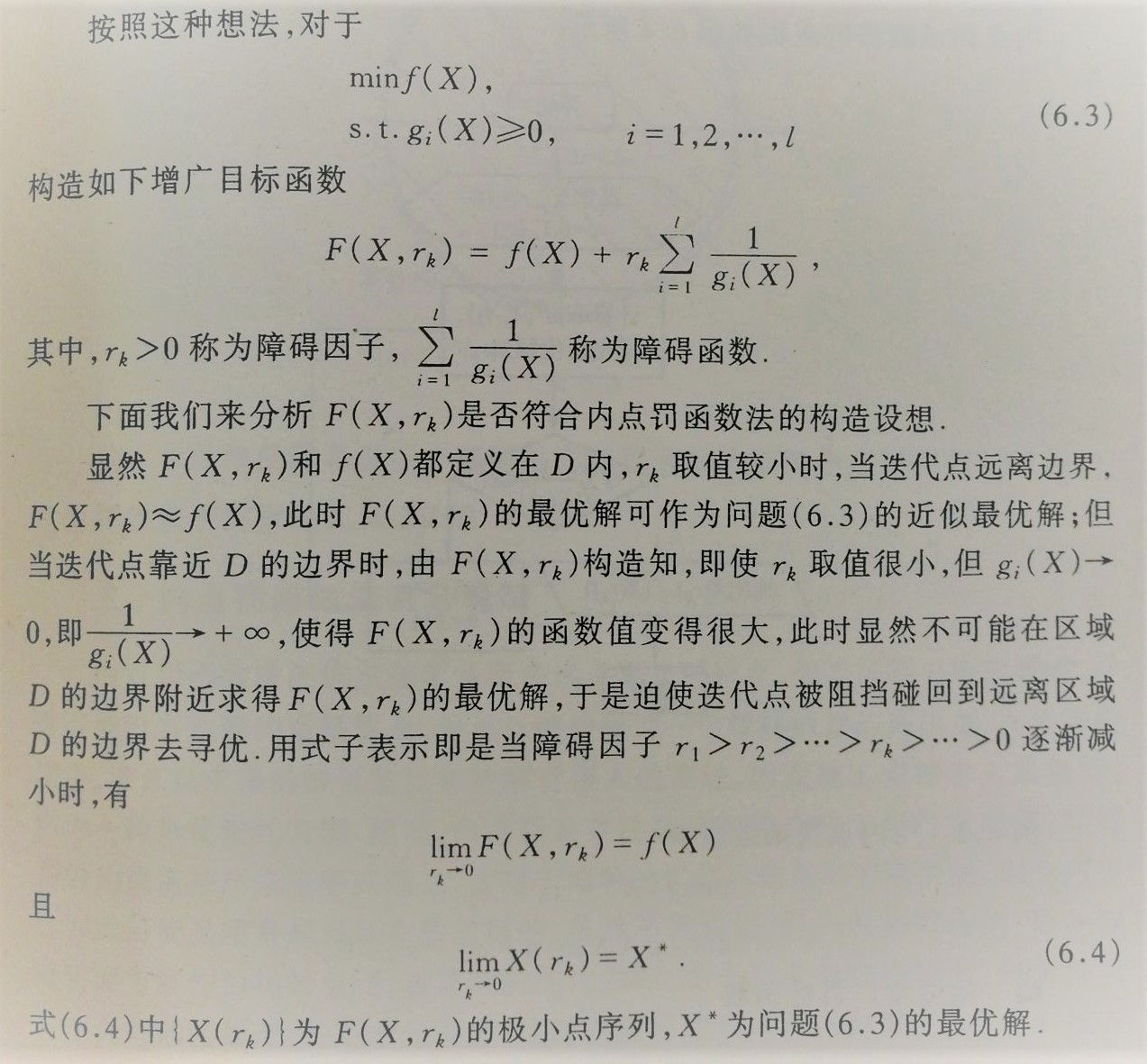特点
该方法保证了迭代过程中得到的解一直处于可行域内，但仍然面临过三个问题：
(1)需要找到一个可行的初始点。
(2)如果搜索步长过大，有可能一步跨过壁垒，所以在搜索过程中应适当控制步长，防止该情况发生。
(3)无法解决等式约束的问题。

3、混合罚函数法

基本思路
为解决内点法不能应对等式约束的问题，采用以下混合罚函数：
F(X,rk)=f(X)+rk∑i=1l1gi(X)+1rk−−√∑j=1m[hj(X)]2$F\left(X,{r}_{k}\right)=f\left(X\right)+{r}_{k}\sum _{i=1}^{l}\frac{1}{{g}_{i}\left(X\right)}+\frac{1}{\sqrt{{r}_{k}}}\sum _{j=1}^{m}\left[{h}_{j}\left(X\right){\right]}^{2}$
F(X,r_k)=f(X)+r_k \sum_{i=1}^l \dfrac{1}{g_i(X)}+\dfrac{1}{\sqrt{r_k}}\sum_{j=1}^m [h_j(X)]^2

外插技术
F(X,r)$F\left(X,r\right)$$F(X,r)$的最优解X∗${X}^{\ast }$$X^*$可以看做r$r$$r$的函数，当我们得到了前几点的X∗(r)${X}^{\ast }\left(r\right)$$X^*(r)$后，可以通过外插法估计下一个最优值，并把该点当做下一次迭代的初始点，以加快收敛速度。
通常使用两点外插或者三点外插。

4、约束坐标轮换法

基本思路
与无约束优化问题的坐标轮换法类似，但是搜索时不再使用一般的以为搜索方法，而采用以下规则：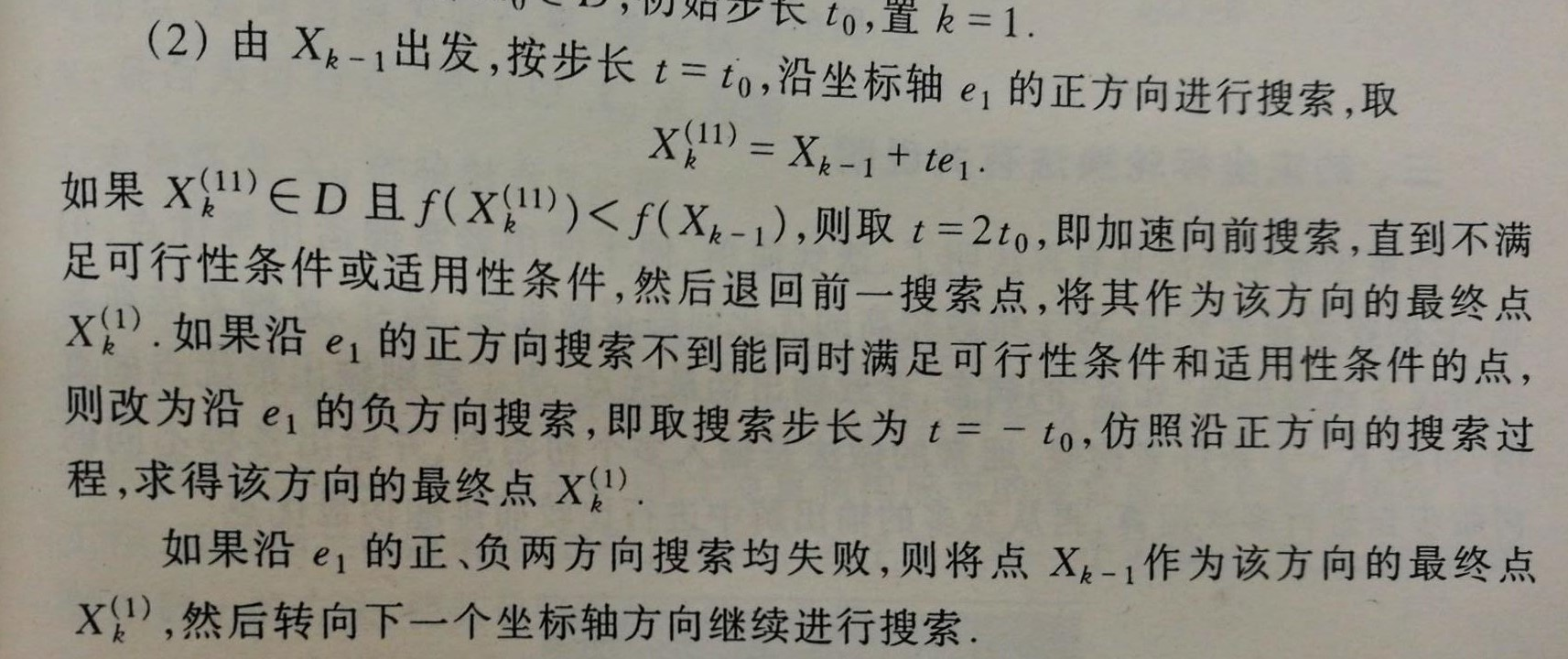该方法可以引入一个步长缩放因子，当在t步长下的搜索已满足该步长下的终止限，而步长并不足够下，则缩短步长进行下一轮搜索。
特点
该方法算法简单明了，但存在以下问题：
(1)收敛较慢；
(2)可能出现“死点”，导致输出伪最优点；
(3)可能出现在某几个点之间循环的现象。

5、复合型法

基本思路
与单纯形法类似，但该方法使用的是顶点更多的复合型。（顶点可以使降维的可能性减小，而且由于约束边界的限制，使用单纯形容易“卡死”在某个地方，而复合型则提供了更多可能的搜索方向）
具体细节
(1)生成初始复合型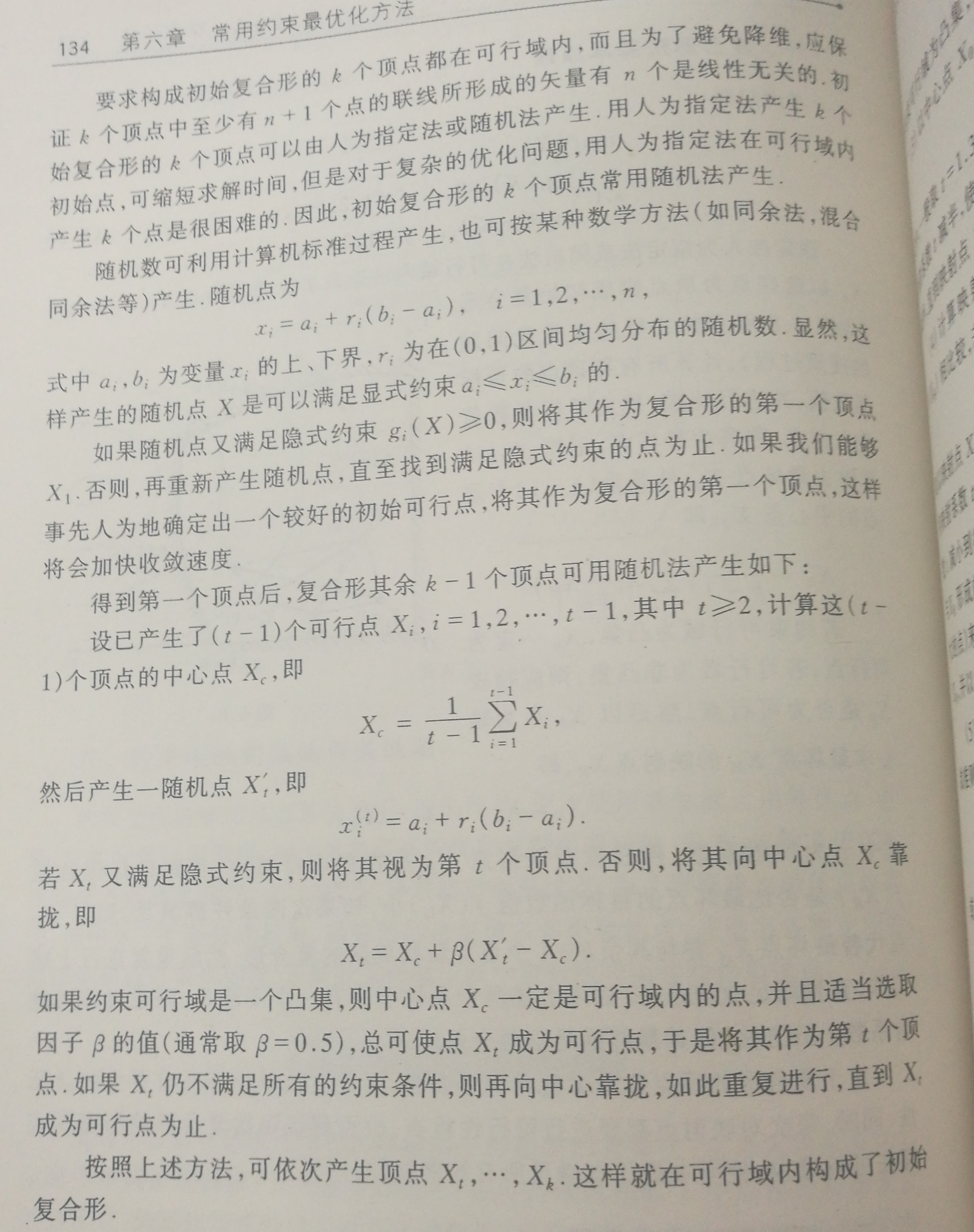(2)跑出可行域的点一律认为是最坏的点。
(3)如果无法利用最坏点搜索（即步长t降的很小仍不符合要求）则改用次坏点替代最坏点，并以此类推。
(4)关于复合型的顶点数，当n≤5$n\le 5$$n \le 5$时，一般取k=2n$k=2n$$k=2n$，n>5$n>5$$n>5$时一般取k=(1.25∼1.5)n$k=\left(1.25\sim 1.5\right)n$$k=(1.25\sim1.5)n$。
特点
属于直接法，对目标函数要求少。
但其收敛速度较慢，而且不能应对等式约束。

展开全文• 最优化方法》复习题 含答案 孙文瑜 徐成贤版（附录 5 《最优化方法》复习题）
• - 最优化方法及其应用/郭科，陈聆，魏友华.-北京：高等教育出版社,2007.7(2013.7重印) 在第四章中，我们解决了确定搜索步长的问题，现在解决确定搜索方向的问题。 1、最速下降法 基本思路 搜索方向总沿着f(X)f...
主要参考书目：

最优化方法及其应用/郭科，陈聆，魏友华.-北京：高等教育出版社,2007.7(2013.7重印)

在第四章中，我们解决了确定搜索步长的问题，现在解决确定搜索方向的问题。

1、最速下降法

基本思路
搜索方向总沿着f(X)$f\left(X\right)$$f(X)$在Xk${X}_{k}$$X_k$点的负梯度方向，因为在Xk${X}_{k}$$X_k$点的某个邻域内，该方向上目标函数下降最快。
即
Pk=−▽f(Xk).${P}_{k}=-▽f\left({X}_{k}\right).$
P_k=-\bigtriangledown f(X_k).

具体细节
运用于正定二次函数可以得到显式递推公式：
Xk+1=Xk−gTkgkgTkAgkgk.${X}_{k+1}={X}_{k}-\frac{{g}_{k}^{T}{g}_{k}}{{g}_{k}^{T}A{g}_{k}}{g}_{k}.$
X_{k+1}=X_k-\dfrac{g^T_k g_k}{g^T_k A g_k}g_k .

特点
该方法虽然叫做“最速下降法”，实际上收敛速度并不快。
其主要原因是每次迭代后下一次的搜索方向总是和前一次相互垂直，产生锯齿现象，使得收敛速度较为缓慢。
锯齿现象如图：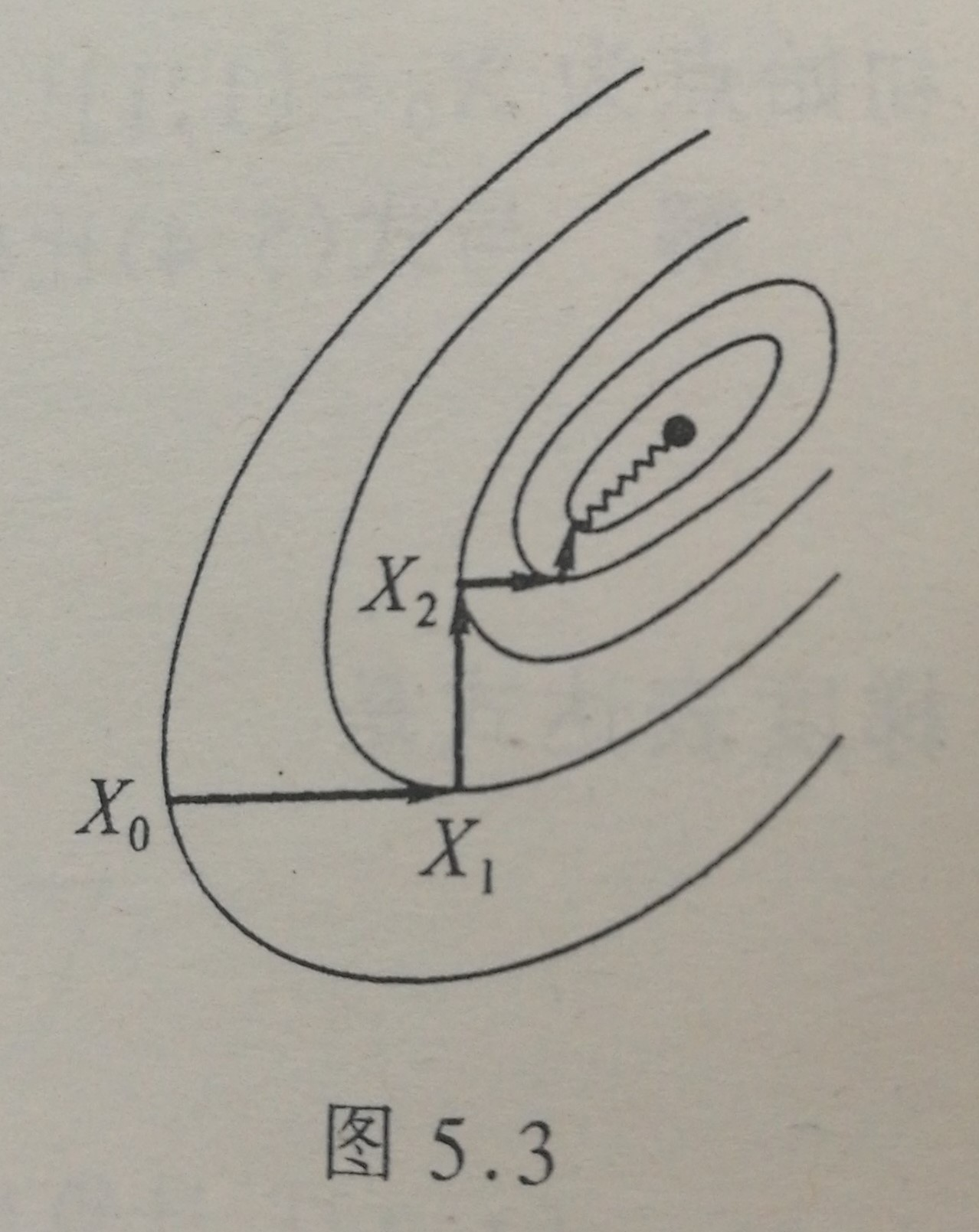2、牛顿法

基本思路
每轮迭代在迭代的起始点Xk${X}_{k}$$X_k$处用一个适当的二次函数来近似该点处的目标函数，由此用Xk${X}_{k}$$X_k$指向近似二次函数极小点的方向来构造搜索方向Pk${P}_{k}$$P_k$。
如图：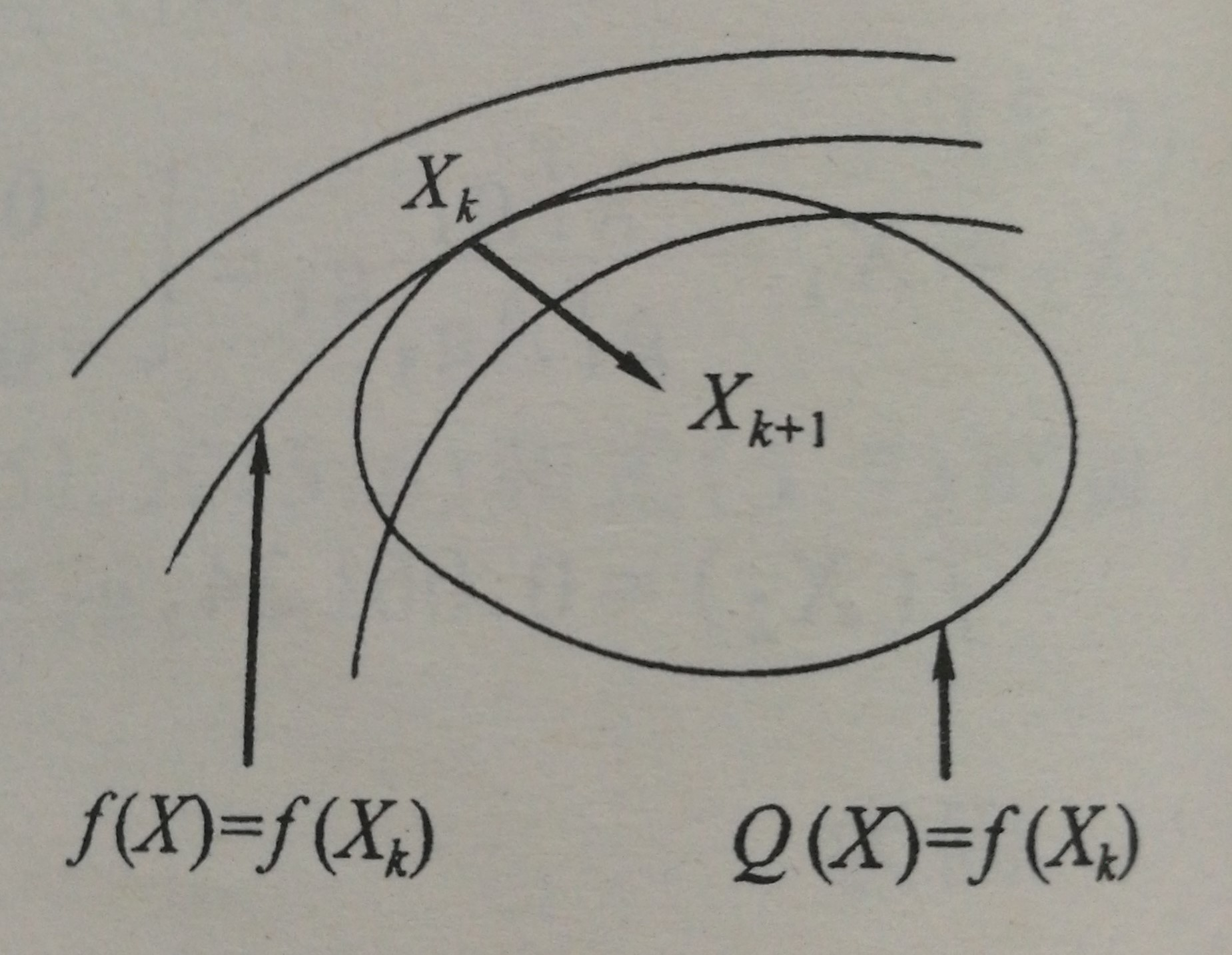具体细节
假设原函数二阶可导且Hesse矩阵正定。
则可以通过在Xk${X}_{k}$$X_k$处进行泰勒展开来寻找近似二次函数，找到该函数后就可以找到指向其极小点的方向Pk${P}_{k}$$P_k$作为搜索方向。而搜索步长tk${t}_{k}$$t_k$恒取1。
其中
Pk=−[▽2f(Xk)]−1▽f(Xk).${P}_{k}=-\left[{▽}^{2}f\left({X}_{k}\right){\right]}^{-1}▽f\left({X}_{k}\right).$
P_k=-[\bigtriangledown ^2 f(X_k)]^{-1} \bigtriangledown f(X_k).

特点
该方法收敛很快，但是：
(1)迭代只能保证函数值不升，而不能保证函数值下降。
(2)要求Hesse矩阵必须正定。
(3)要求初始点比较接近极值点或者有利于接近极值点。
(4)当Hesse矩阵奇异时，无法求出搜索方向。
(5)计算内存需求较大。

3、修正牛顿法

基本思路
保留牛顿法确定的搜索方向，同时用一维搜索来确定步长。
特点
继承了牛顿法优点的基础上，对初始点的要求不再那么严格了。但牛顿法的其他缺陷未能得到克服。

4、共轭方向法特别地，当Q为单位矩阵时，称d(1),d(2)${d}^{\left(1\right)},{d}^{\left(2\right)}$$d^{(1)},d^{(2)}$正交。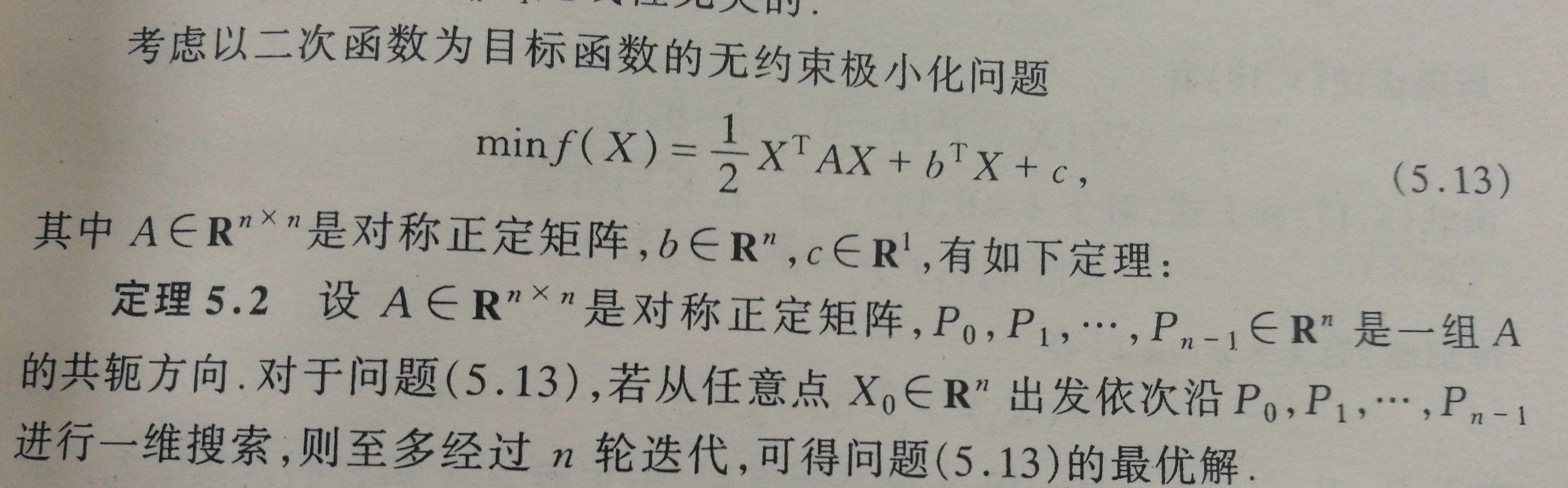以上这种从任意点出发，沿某组共轭方向进行一维搜索的方法称为共轭方向法。
一般地，在n维空间可以找到n个互相共轭的方向，故而对于n元正定二次函数，利用共轭方向法总可以在n次迭代内找到极小点。
对于n元正定二次目标函数，从任意初始点出发，如果经过有限次迭代就可以求得极小值，则称这种算法具有二次终止性。
一般来说，具有二次终止性的算法在应用于一般函数时，收敛速度较快。
至此，只是叙述了共轭方向法的基本思路，尚未言明如何寻找共轭方向。以下将介绍共轭梯度法

5、共轭梯度法

基本思路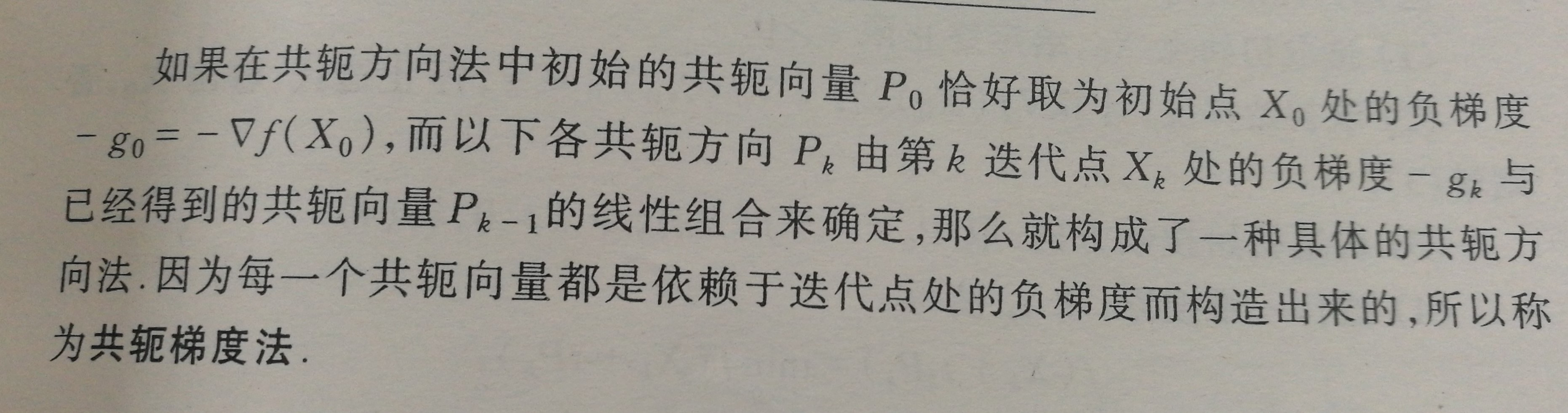具体细节
设
Pk+1=−▽f(Xk+1)+λkPk,  k=0,1,2,⋯,n−2.
P_{k+1}=-\bigtriangledown f(X_{k+1})+\lambda_kP_k,\ \ k=0,1,2,\cdots,n-2.

通过计算可以确定：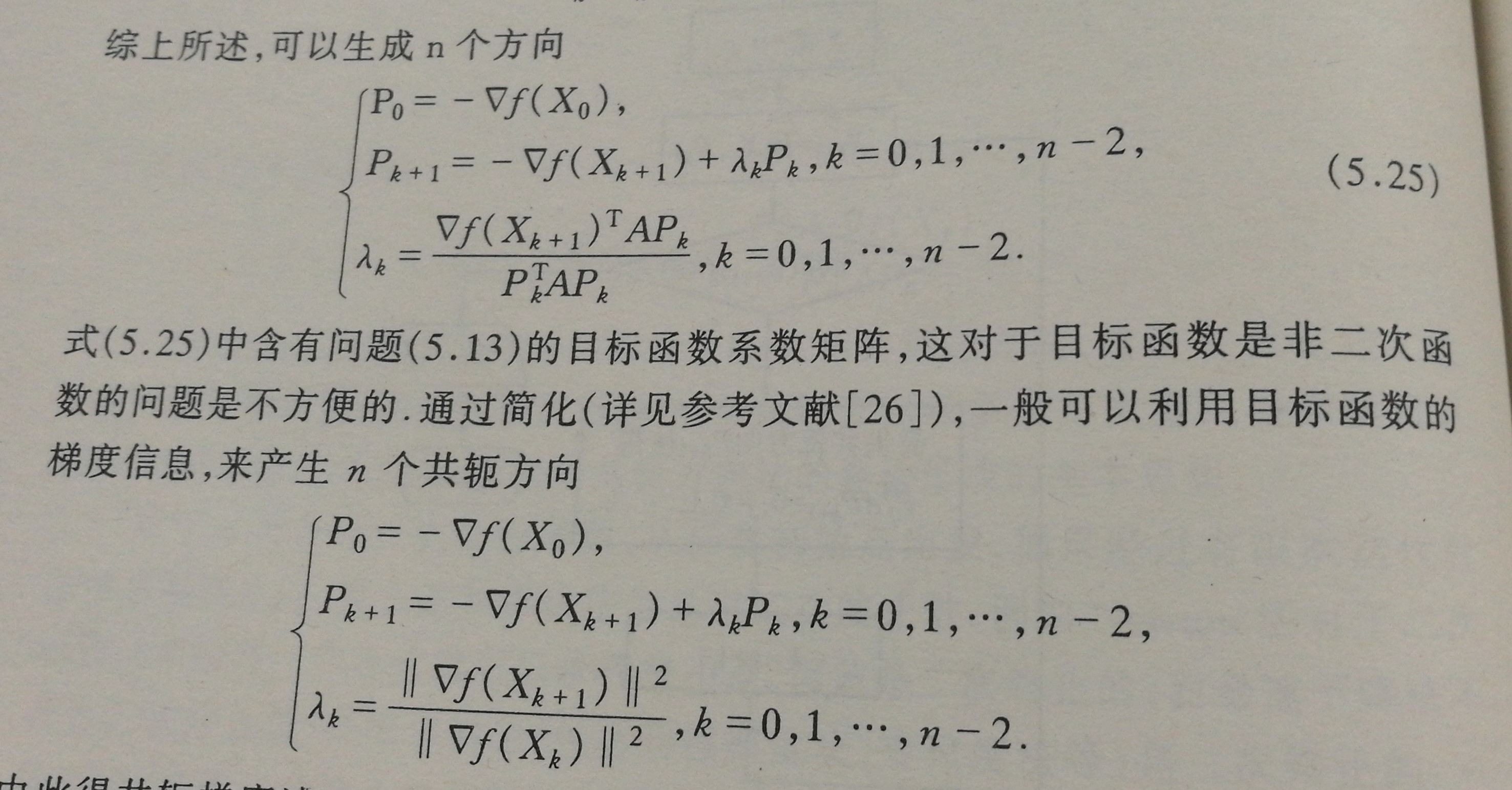因为实际的目标函数并不一定是正定二次函数，所以在进行一轮迭代（就是每个方向都进行一次）后不一定能取得极小点。此时，我们令X0=Xk${X}_{0}={X}_{k}$$X_0=X_k$再进行下一轮迭代即可。同时这样也克服了因舍入误差导致向量不再共轭的问题。
特点
共轭梯度法可以看做最速下降法的一种改进（λk=0${\lambda }_{k}=0$$\lambda_k=0$即是最速下降法），收敛较快，与牛顿法相比则不必计算Hesse矩阵，对目标函数的性质要求较少，计算所需内存较少。

6、变尺度法

基本思路
保持牛顿法收敛快的优点，同时避免Hesse矩阵的计算。
牛顿法的迭代公式为：
Xk+1=Xk−G−1kgk,k=0,1,2,⋯${X}_{k+1}={X}_{k}-{G}_{k}^{-1}{g}_{k},k=0,1,2,\cdots$
X_{k+1}=X_k-G^{-1}_kg_k,k=0,1,2,\cdots

这里可以通过某种近似矩阵Hk${H}_{k}$$H_k$来代替G−1k${G}_{k}^{-1}$$G^{-1}_k$,同时考虑到搜索步长，迭代公式变为：
Xk+1=Xk−tkHkgk,${X}_{k+1}={X}_{k}-{t}_{k}{H}_{k}{g}_{k},$
X_{k+1}=X_k-t_kH_kg_k,

Hk${H}_{k}$$H_k$需要满足一些条件：
(1)Hk${H}_{k}$$H_k$正定，保证了迭代公式具有下降性质。
(2)Hk${H}_{k}$$H_k$之间具有简单迭代形式，如Hk+1=Hk+Ek${H}_{k+1}={H}_{k}+{E}_{k}$$H_{k+1}=H_k+E_k$，其中Ek${E}_{k}$$E_k$称为校正矩阵，该公式称为校正公式。
(3)Hk${H}_{k}$$H_k$必须满足拟牛顿条件：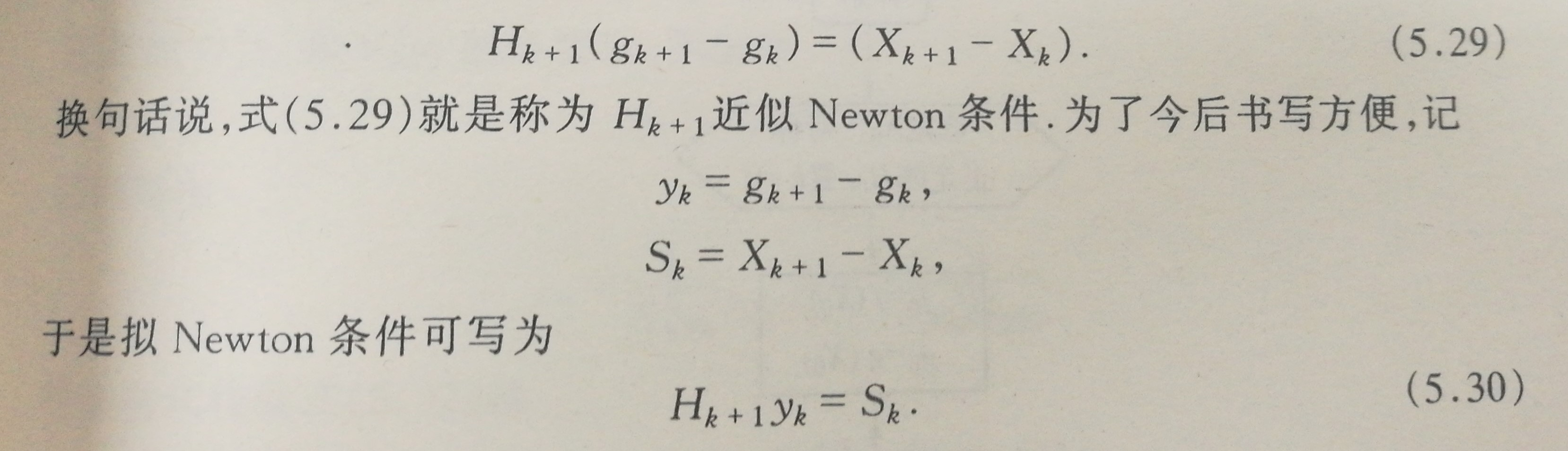其中g$g$$g$为梯度。
变尺度法的不同即是校正矩阵的不同，以下介绍两种常用算法：
DFP算法
Hk+1=Hk+SkSTkSTkyK−HkykyTkHkyTkHkyk.${H}_{k+1}={H}_{k}+\frac{{S}_{k}{S}_{k}^{T}}{{S}_{k}^{T}{y}_{K}}-\frac{{H}_{k}{y}_{k}{y}_{k}^{T}{H}_{k}}{{y}_{k}^{T}{H}_{k}{y}_{k}}.$
H_{k+1}=H_k+\dfrac{S_kS_k^T}{S_k^Ty_K}-\dfrac{H_k y_k y_k^T H_k}{y_k^T H_k y_k}.

BFGS算法
考虑形式：
Hk+1=Hk+SkSTkyTkSK−HkykyTkHkyTkHkyk+β(yTkSk)(yTkHkyk)WKWTk,${H}_{k+1}={H}_{k}+\frac{{S}_{k}{S}_{k}^{T}}{{y}_{k}^{T}{S}_{K}}-\frac{{H}_{k}{y}_{k}{y}_{k}^{T}{H}_{k}}{{y}_{k}^{T}{H}_{k}{y}_{k}}+\beta \left({y}_{k}^{T}{S}_{k}\right)\left({y}_{k}^{T}{H}_{k}{y}_{k}\right){W}_{K}{W}_{k}^{T},$
H_{k+1}=H_k+\dfrac{S_kS_k^T}{y_k^TS_K}-\dfrac{H_k y_k y_k^T H_k}{y_k^T H_k y_k}+\beta (y_k^TS_k) (y_k^TH_ky_k)W_KW_k^T,

其中
Wk=SkyTkSk−HkykyTkHkyk.${W}_{k}=\frac{{S}_{k}}{{y}_{k}^{T}{S}_{k}}-\frac{{H}_{k}{y}_{k}}{{y}_{k}^{T}{H}_{k}{y}_{k}}.$
W_k=\dfrac{S_k}{y_k^TS_k}-\dfrac{H_ky_k}{y_k^TH_ky_k}.

可见，当β=0$\beta =0$$\beta=0$时，即为DFP算法。
而当β=1STkyk$\beta =\frac{1}{{S}_{k}^{T}{y}_{k}}$$\beta=\dfrac{1}{S_k^T y_k}$时，即是BFGS算法，其校正公式为：
Hk+1=Hk+1STkyk[(1+yTkHkykSTkyk)SkSTk−HkyKSTk−SkyTkHk]${H}_{k+1}=\phantom{\rule{0ex}{0ex}}{H}_{k}+\frac{1}{{S}_{k}^{T}{y}_{k}}\left[\left(1+\frac{{y}_{k}^{T}{H}_{k}{y}_{k}}{{S}_{k}^{T}{y}_{k}}\right){S}_{k}{S}_{k}^{T}-{H}_{k}{y}_{K}{S}_{k}^{T}-{S}_{k}{y}_{k}^{T}{H}_{k}\right]$
H_{k+1}=\\
H_k+\dfrac{1}{S_k^T y_k}[(1+\dfrac{y_k^TH_ky_k}{S_k^T y_k})S_kS_k^T-H_ky_KS_k^T-S_ky_k^TH_k]

特点
对于DFP算法，由于一维搜索的不精确和计算误差的累积可能导致某一轮的Hk${H}_{k}$$H_k$奇异，而BFGS法则不是很容易发生此种情况。BFGS算法比DFP算法更具有好的数值稳定性。

7、坐标轮换法

基本思路
依次在每个维度进行一维搜索。
假设目标函数为f(x,y)$f\left(x,y\right)$$f(x,y)$，则依次在[1,0],[0,1]$\left[1,0\right],\left[0,1\right]$$[1,0],[0,1]$方向进行搜索。
特点
算法简单，但计算效率较低。

8、单纯形法

基本思路
对于n维问题，在n维空间中选择n+1个点作为顶点构成n维单纯形，找到这n+1个点中最差的点，并向其反方向搜索得到一个新点，与其余n个点构成新的单纯形。知道满足收敛条件。
具体细节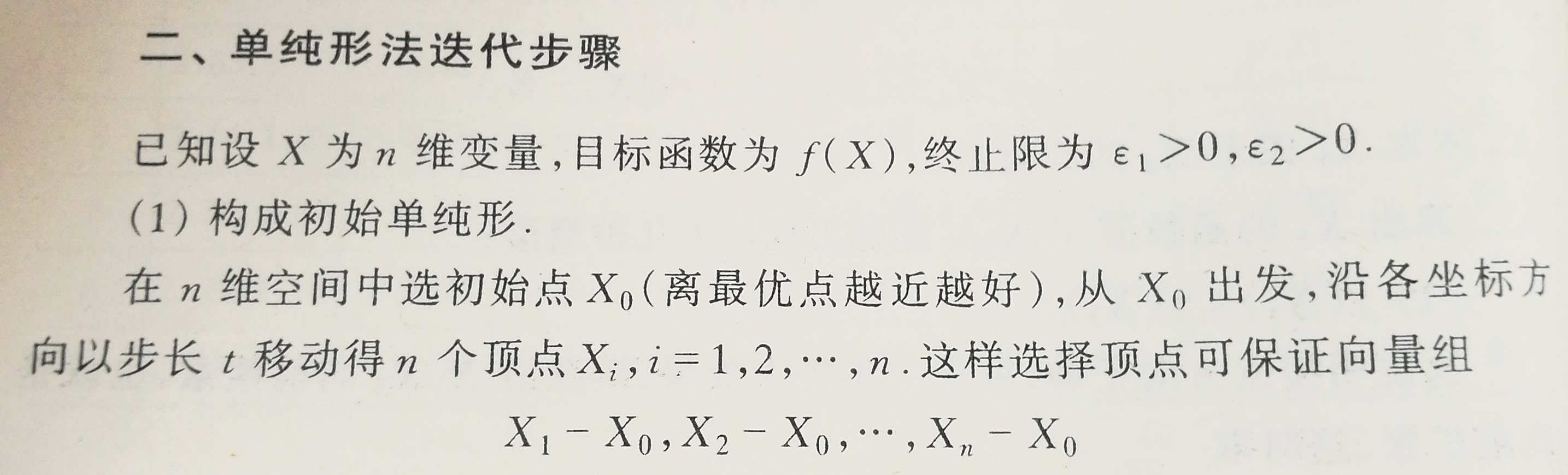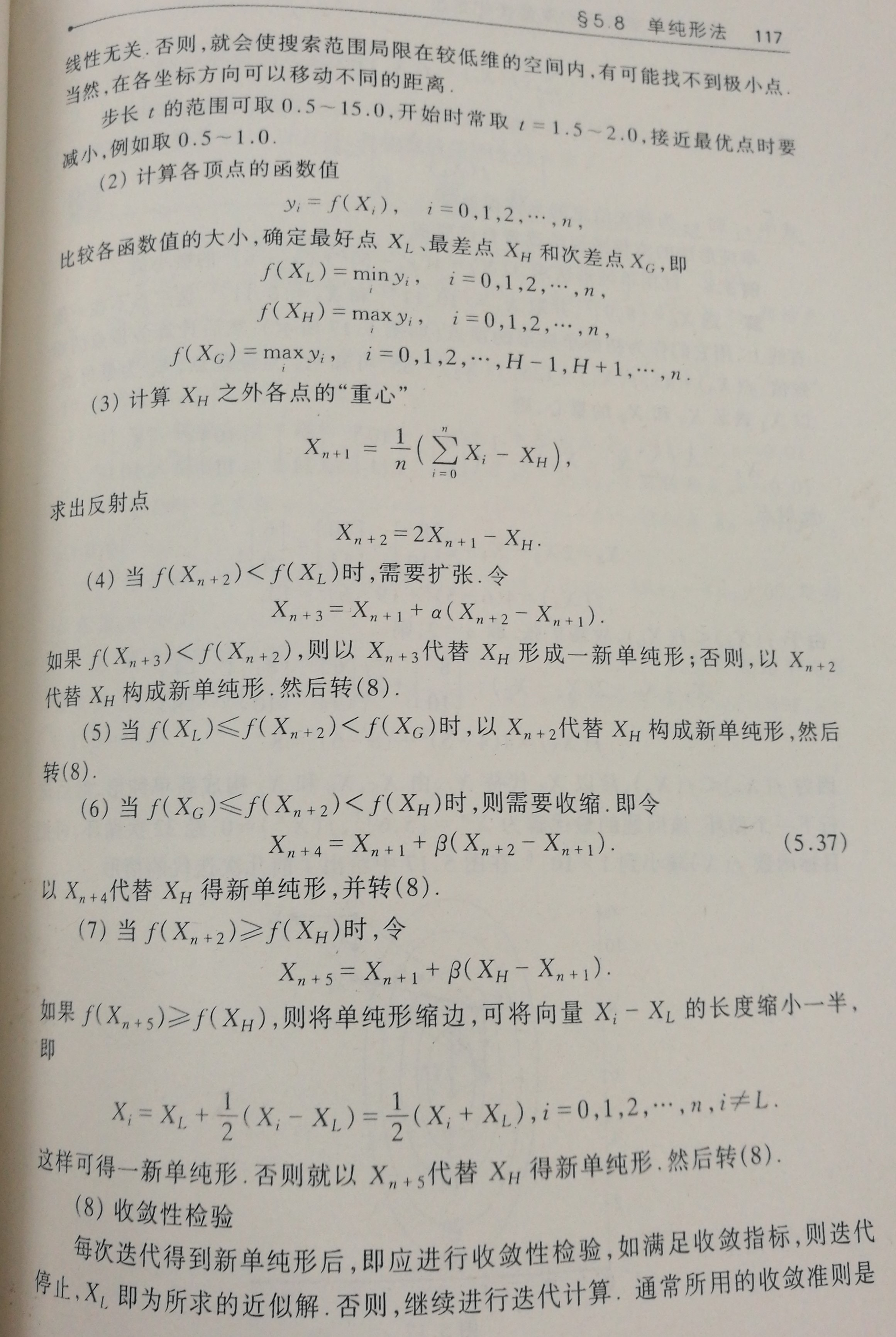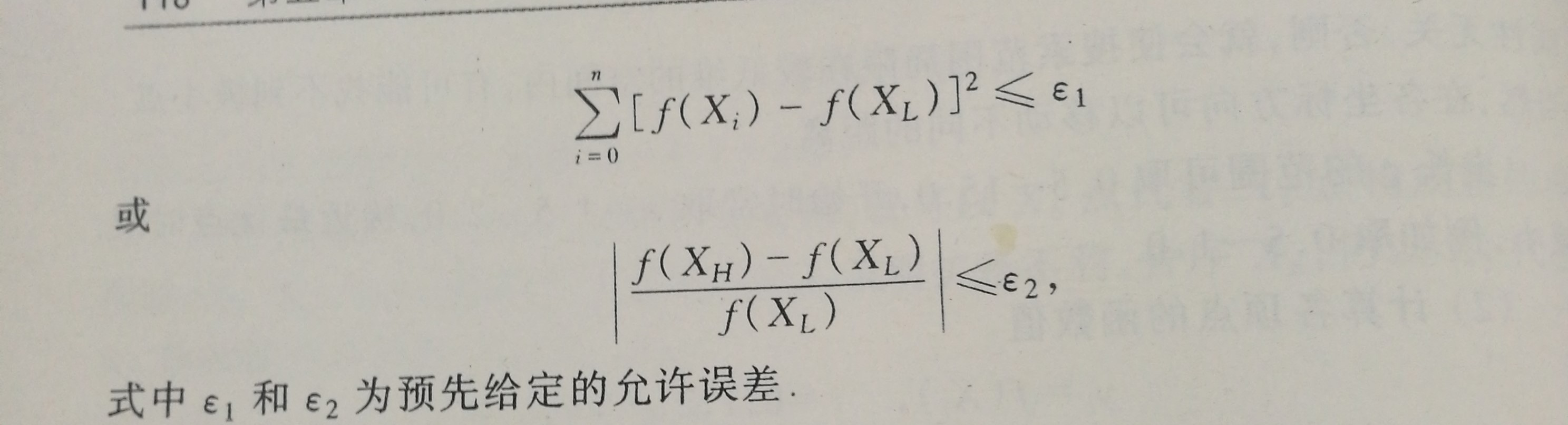特点
该方法占用内存很少，可以方便的求解一些精度要求较低的问题。但对于维度较高（>10）的问题不是很有效。

展开全文• 本文介绍了无约束最优化的两种常用方法，梯度法和牛顿法，拟牛顿法和BFGS，Broyden类算法
梯度下降法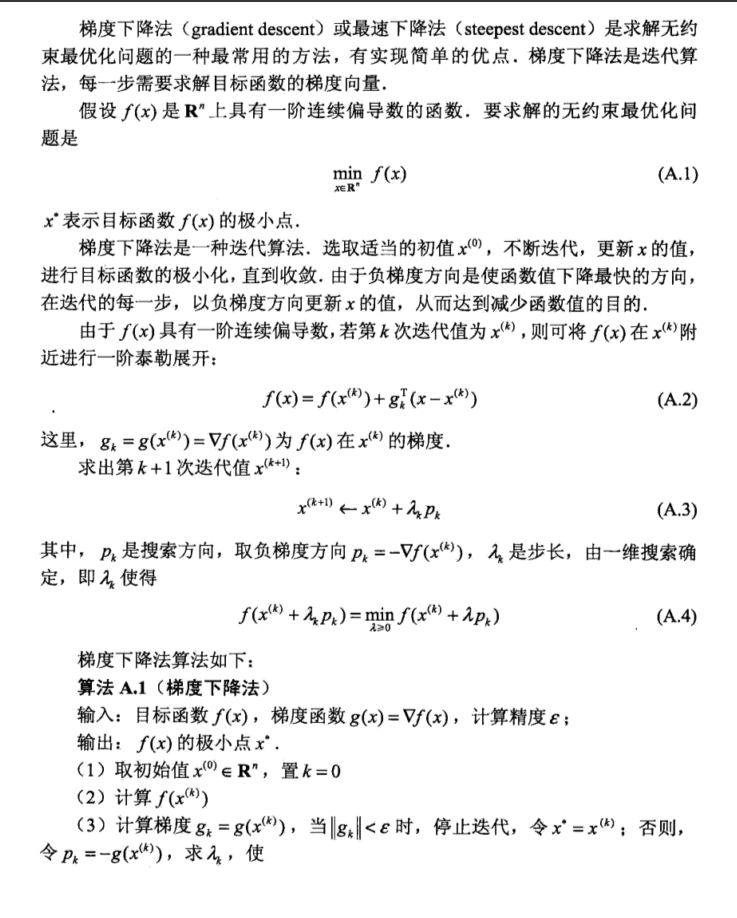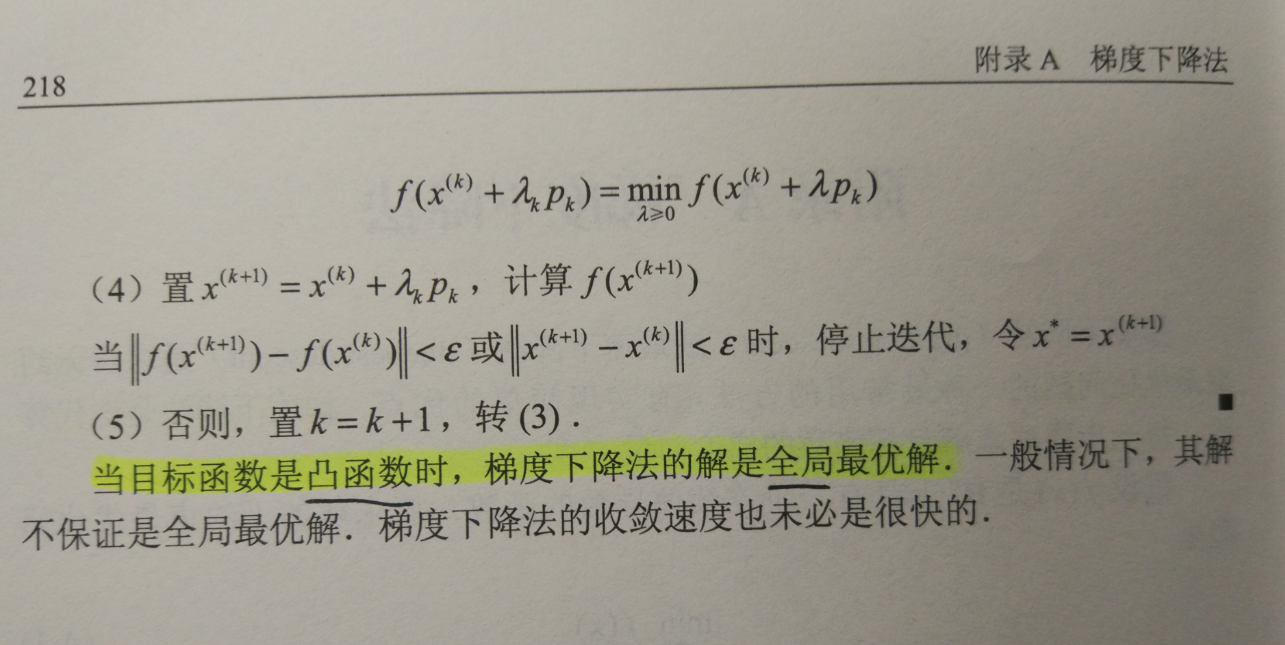牛顿法

牛顿法要求函数具有二阶连续偏导数，利用了函数在极小值点处一阶偏导数为0的必要条件进行优化。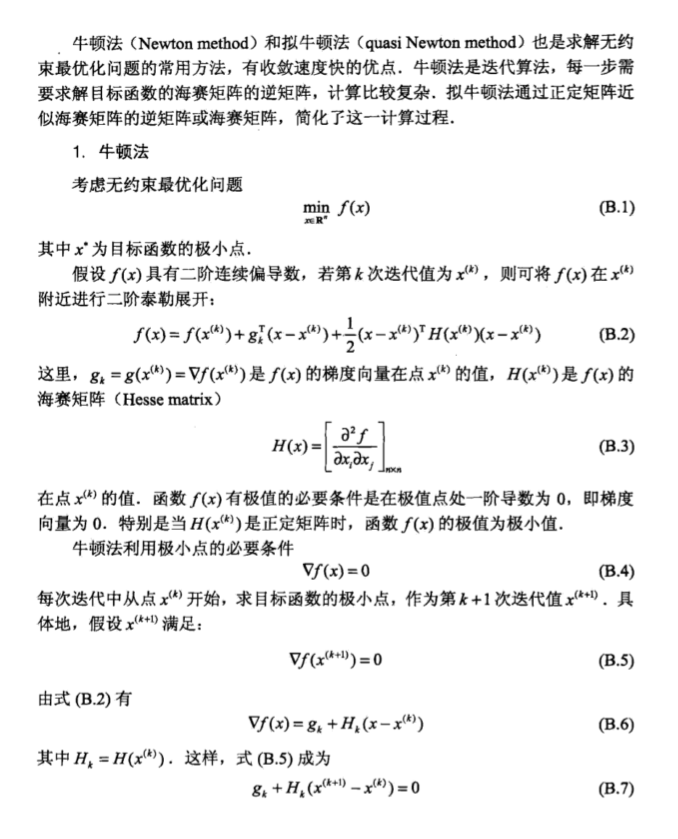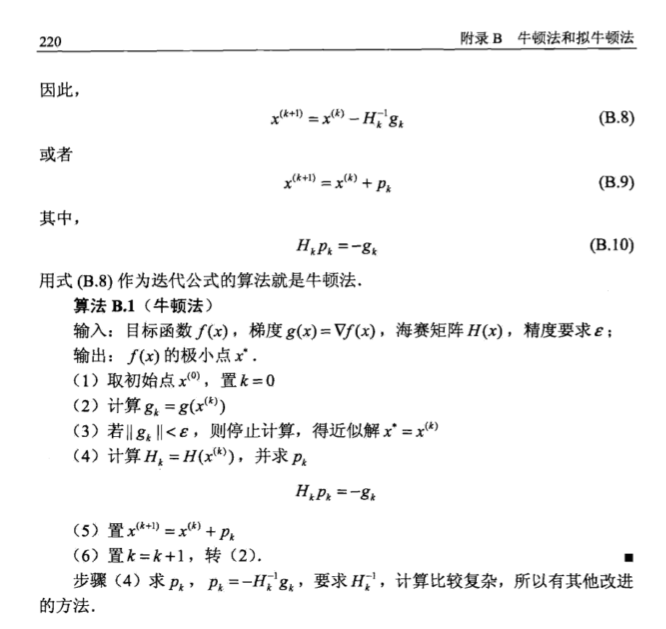拟牛顿法

基本思想
在牛顿迭代法中，需要计算Hessian矩阵的的逆矩阵H−1<!--//--><![CDATA[//><!--
H^{-1}
//--><!]]>，这一计算比较复杂，考虑用一个n阶矩阵Gk=G(x(k))<!--//--><![CDATA[//><!--
G_k=G(x^{(k)})
//--><!]]>来近似代替H−1k=H−1(x(k))<!--//--><![CDATA[//><!--
H_k^{-1}=H^{-1}(x^{(k)})
//--><!]]>。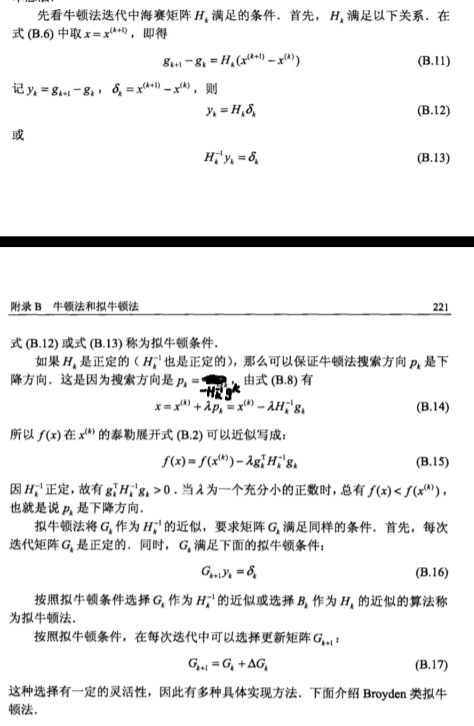BFGS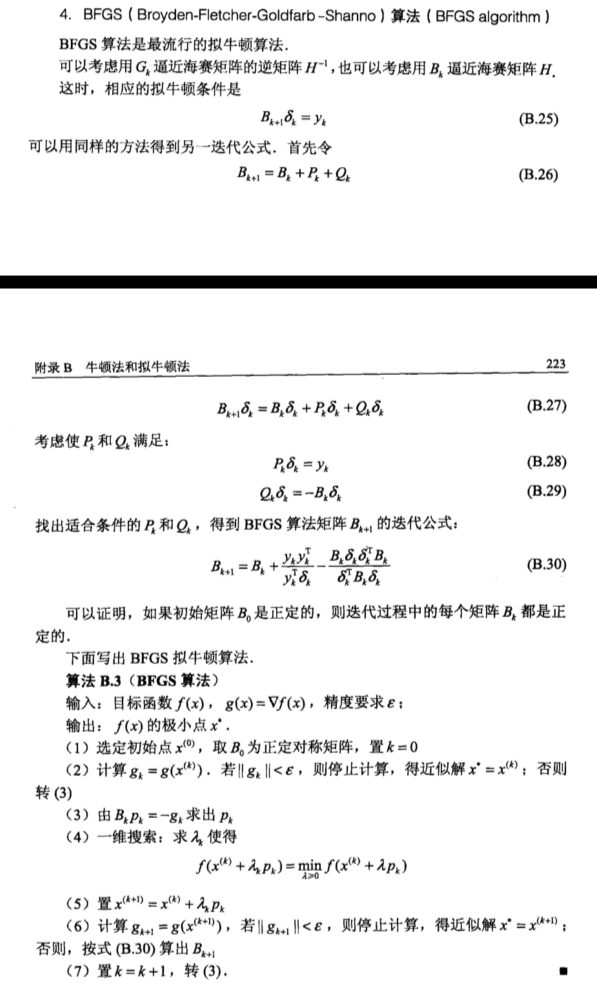Broyden类算法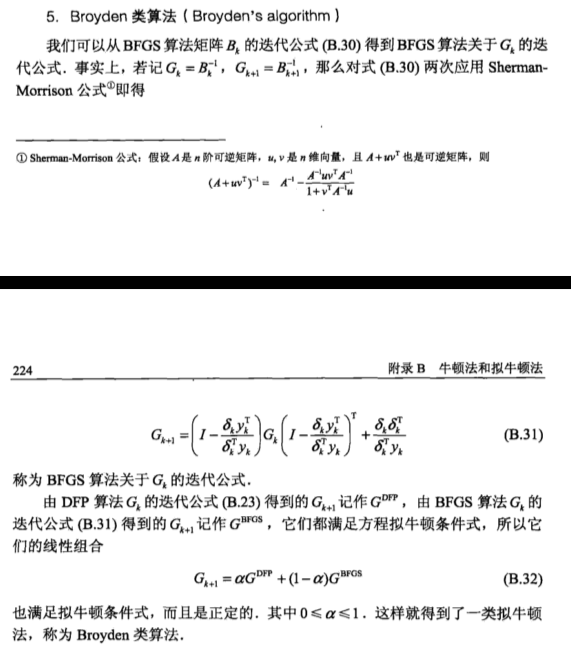参考资料

《统计学习方法》 附录

展开全文• 数值最优化方法 [高立] 2014年版，北京大学出版，压缩包解压为PDF扫描版。数学
• ## 最优化方法：拉格朗日乘数法

万次阅读 多人点赞 2016-08-18 14:34:38
1. 拉格朗日乘数法的基本思想　作为一种优化算法，拉格朗日乘子法主要用于解决约束优化问题，它的基本思想就是通过引入拉格朗日乘子来将含有n个变量和k个约束条件的约束优化问题转化为含有（n+k）个变量的无约束优化...拉格朗日乘数法
• ## 无约束最优化方法学习笔记

万次阅读 多人点赞 2015-08-11 23:12:24
这一段时间学习优化理论中的一些经典方法，写下一些要点和体会，帮助自己和有需要的人理解最优化方法。１.基础知识首先来看无约束最优化问题： minf(x)\begin{equation} \min f(x) \end{equation} 其中函数 f:Rn...共轭梯度法 最小二乘法
• 最优化方法及其Matlab程序设计》较系统地介绍了非线性最优化问题的基本理论和算法，以及主要算法的Matlab程序设计，主要内容包括（精确或非精确）线搜索技术、最速下降法与（修正）牛顿法、共轭梯度法、拟牛顿法、...
• 一本关于最优化算法的书，对于做设计和算法有指导意义！希望抛砖引玉——程序！
• ## 数值最优化方法

千次阅读 2017-07-28 11:41:54
算法来源：《数值最优化方法~高立》 算法目的：实现函数的局部最优化寻找，以二元函数为例，展示了最速下降法和牛顿寻优的算法过程 主要Python模块：numpy,sympy （1）Python实现 （2）MATLAB实现 ...
• ## 常用最优化方法

千次阅读 2018-05-18 14:14:15
熟悉机器学习的童鞋都知道，优化方法是其中一个非常重要的话题，最常见的情形就是利用目标函数的导数通过多次迭代来求解无约束最优化问题。实现简单，coding方便，是训练模型的必备利器之一。 2. 几个数学概念 1)...
•非线性规划 梯度下降法
• 华电北风吹 天津大学认知科学与计算重点实验室 日期 2015/11/7本文主要从试探法、插值法、微积分中的求根法讲解怎么求解一维函数极值问题。 一、试探法 1、斐波那契法(分数法) 适用条件：存在极值点的一维凸...
• 本文是Deep Learning 之 最优化方法系列文章的RMSProp方法。主要参考Deep Learning 一书。 整个优化系列文章列表： Deep Learning 之 最优化方法 Deep Learning 最优化方法之SGD Deep Learning 最优化方法之...
• 本文是Deep Learning 之 最优化方法系列文章的Adam方法。主要参考Deep Learning 一书。 整个优化系列文章列表： Deep Learning 之 最优化方法 Deep Learning 最优化方法之SGD Deep Learning 最优化方法之...
• 本文是Deep Learning 之 最优化方法系列文章的SGD方法。主要参考Deep Learning 一书。 整个优化系列文章列表： Deep Learning 之 最优化方法 Deep Learning 最优化方法之SGD Deep Learning 最优化方法之...
• http://blog.csdn.net/pipisorry/article/details/23553263非线性方程求根方法中的数学定义零点1 如果 x * 使 f( x * )=0,则称 x * 为方 程的根,或称为 函数f( x )的零点;当 f( x )为多项式时,即其中0这里我们要求...数值分析 二分法 不动点迭代...